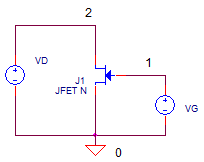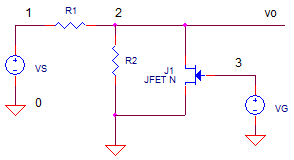eCircuit  Center

### JFET Variable Resistor

CIRCUITSome designs call for a variable resistor such as variable gain amplifiers, attenuators and some guitar effect boxes. The main goal here is device whose resistance is some function of bias voltage. One such device is your basic JFET. For small signals, where the device operates in the linear (or ohmic) region, the resistance between the Drain and Source terminals (Rds) decreases as the gate voltage increases.

IV CURVES
How can you determine the JFET resistance versus bias voltage? Nothing tells the story like the slope of the IV curve. Here's the basic circuit to develop these insightful graphs.

* GATE AND DRAIN VOLTAGE
VG 1 0 DC 0
VD 2 0 DC 0
J1 2 1 0 J2N5952
*
* Nested DC sweep
.DC VD -0.05 0.05 0.1 VG -1.75 0 0.25

The file includes an essential line - the nested DC sweep command.

.DC V1 Start Stop Incr V2 Start Stop Incr

You define how the test voltages V1 and V2 will start, stop and increment. Then, for each V1 voltage point, V2 will sweep through its entire range from start to stop. This comes in handy where the drain (VD) is swept through its range for every gate bias voltage (VG).

CIRCUIT INSIGHT   Run a DC analysis of the 2N5952 JFET in the JFET_VARIABLE_RES1.CIR and plot the drain current ID(J1). The drain is only swept from -50mV to +50mV. You should see a nice family of curves representing the Id as a function of Vd for each value of Vg. The slope of each VI curve represents the Drain-Source resistance. What is the minimum resistance? The smallest slope (resistance) can be approximated by Rds = 50mV/30uA = 1.66k ohms. The largest slope is Rds = 50mV/250uA = 200 ohms. This shows the range of resistance possible when sweeping the gate from -1.75 to 0V.

As warned earlier, this resistor-like operation applies only to small signals. What about larger signals? Increase the Vd sweep parameters to Start = -0.5V and Stop = +0.5V. What happens to the linearity of the IV plots?

VARIABLE ATTENUATOR
Here's an application of a JFET taken straight out of a National app note. The JFET implements a voltage attenuator as one leg in a voltage divider.

The voltage divider ratio is defined by Vo/Vs = R2'/(R1+R2') where R2' is actually the parallel combo of R2 and Rds. For this example, setting R1=10k and R2=100k defines a small attenuation, even when the JFET is OFF (very high resistance)

CIRCUIT INSIGHT   The gate voltage VG ramps from -2V to 0V while a 100mV (1kHz) sine wave is applied to the divider input. Run a TRANSIENT ANALYSISs of JFET_VARIABLE_ATTEN1.CIR and plot both the gate voltage (V3) and the output voltage V(2). At what gate voltage does Rds begin conducting and attenuating the signal? Where does the max attenuation occur? You can estimate Rds by measuring V(2) peaks and solving the divider equation for R2.

UPCOMING TOPICS
In a future topic, we'll use the variable resistor as part of a popular guitar effects pedal called the phase shifter.

SPICE FILES

Variable Resistor

Download the file or copy this netlist into a text file with the *.cir extension.

```* JFET_VARIABLE_RES1.CIR
*
* GATE AND DRAIN VOLTAGE
VG  1 0       DC 0
VD  2 0       DC 0
*
J1  2 1 0 J2N5952
*
* Nested DC sweep
.DC  VD -0.05 0.05 0.1   VG -1.75 0 0.25
*
*
.model J2N5952	NJF(Beta=1.314m Betatce=-.5 Rd=1 Rs=1 Lambda=4m Vto=-2.021
+ Vtotc=-2.5m Is=33.57f Isr=322.4f N=1 Nr=2 Xti=3 Alpha=311.7u
+ Vk=243.6 Cgd=1.6p M=.3622 Pb=1 Fc=.5 Cgs=2.414p Kf=10.14E-18 Af=1)
*
.PROBE
.END```

Download the file or copy this netlist into a text file with the *.cir extension.

Variable Attenuator

```* JFET_Variable_Atten1.CIR
*
VS	1	0	SIN(0 0.1V 1000Hz)
R1	1	2	10k
R2	2	0	100k
*
J1	2	3	0	J2N5952
VG	3	0	PWL(0ms -2.5V   100ms 0V)
*
.model J2N5952	NJF(Beta=1.314m Betatce=-.5 Rd=1 Rs=1 Lambda=4m Vto=-2.021
+ Vtotc=-2.5m Is=33.57f Isr=322.4f N=1 Nr=2 Xti=3 Alpha=311.7u
+ Vk=243.6 Cgd=1.6p M=.3622 Pb=1 Fc=.5 Cgs=2.414p Kf=10.14E-18 Af=1)
*
* ANALYSIS *************************************
.TRAN 	0.1MS  100MS
.PROBE
.END```

Top ?